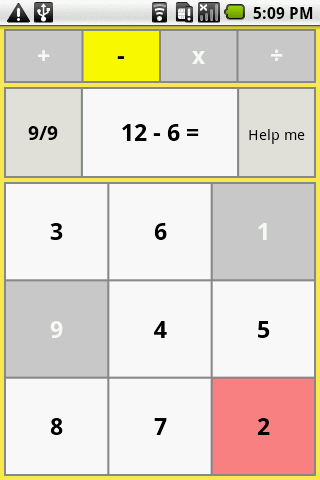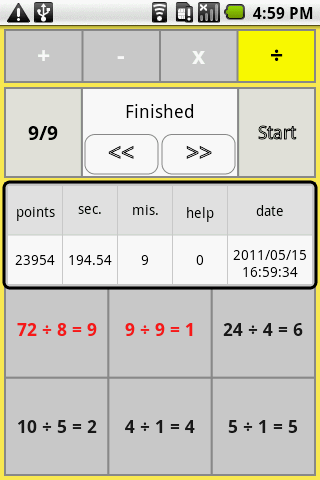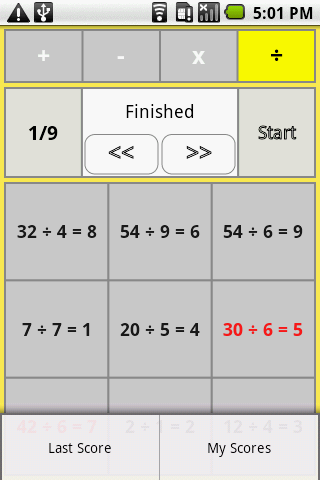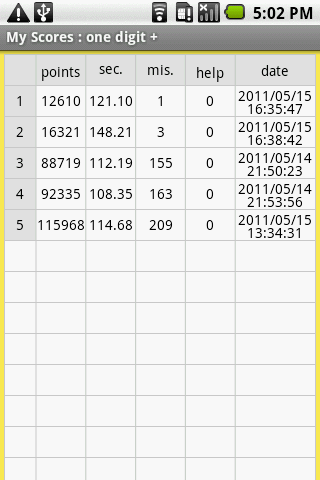Currach Software Mobile / Android Japanese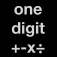one digit +-x÷ Version 1.1 Android Currach Software Mobileupdate 27.Nov.2011

 This App "one digit +-x÷" is one digit arithmetic training App. And this is a searching game, too. This App has four categories, addition:"one digit +" subtraction:"one digit -" multiplication:"one digit x" and division:"one digit ÷". Each category has 9 questions on nine stages, random 81 questions. "one digit +" : from "1 + 1 = 2" to "9 + 9 = 18". "one digit -" : from "2 - 1 = 1" to "18 - 9 = 9". "one digit x" : from "1 x 1 = 1" to "9 x 9 = 81". "one digit ÷" : from "1 ÷ 1 = 1" to "81 ÷ 9 = 9".